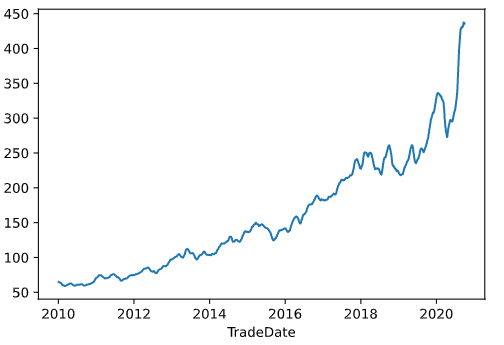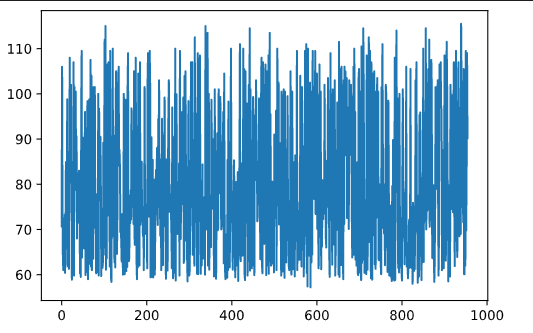DAY 20
0
Microsoft Azure

，簡稱均線。

# 如何製作均線？

## 建立jupyter notebook

``````import pandas
import pyodbc
import matplotlib.pyplot as plt
``````

## 讀檔案

``````with pyodbc.connect(
) as conn:
symbol = "2330"
with conn.cursor() as cursor:
query = f"SELECT TradeDate, ClosePrice FROM [dbo].[DailyPrice] WHERE Symbol ='{symbol}' ORDER BY TradeDate;"
``````

## 製作圖表

``````df.plot()
````````````# 近20日收盤
c_price_20 = df.rolling(20, min_periods=1).mean()
c_price_20.plot()
``````# 後記